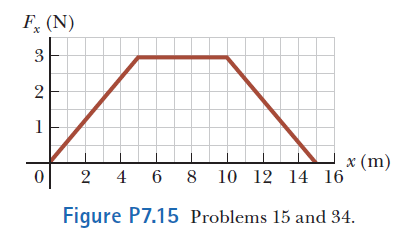# Problem: A particle is subject to a force Fx that varies with position as shown in Figure P7.15. Find the work done by the force on the particle as it moves (a) from x = 0 to x = 5.00 m, (b) from x = 5.00 m to x = 10.0 m, and (c) from x = 10.0 m to x = 15.0 m. (d) What is the total work done by the force over the distance x = 0 to x = 15.0 m?

###### FREE Expert Solution

The work done by the force:

$\overline{){\mathbf{W}}{\mathbf{=}}{{\mathbf{\int }}}_{{\mathbf{x}}_{\mathbf{1}}}^{{\mathbf{x}}_{\mathbf{2}}}{\mathbf{F}}{\mathbf{\left(}}{\mathbf{x}}{\mathbf{\right)}}{\mathbf{d}}{\mathbf{x}}}$

83% (217 ratings)###### Problem Details

A particle is subject to a force Fx that varies with position as shown in Figure P7.15. Find the work done by the force on the particle as it moves (a) from x = 0 to x = 5.00 m, (b) from x = 5.00 m to x = 10.0 m, and (c) from x = 10.0 m to x = 15.0 m. (d) What is the total work done by the force over the distance x = 0 to x = 15.0 m?